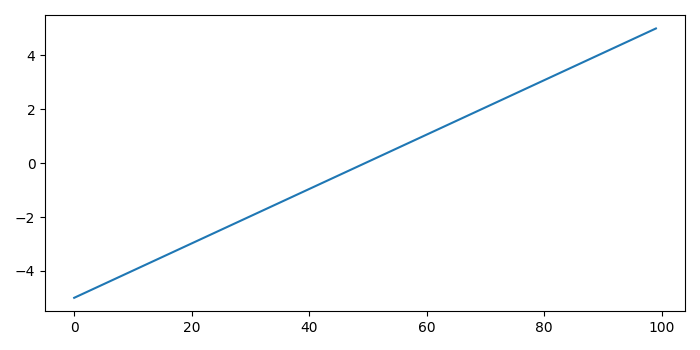# When is plt.Show() required to show a plot and when is it not?

plt.Show() would help whenever there is no interactive plot.

fig.Show() would help to display all the figures if it is interactive.

Let's take an example to observe the difference between plt.Show() and fig.Show().

## Steps

• Open iPython shell.

• Set the figure size and adjust the padding between and around the subplots.

• Create a new figure or activate an existing figure.

• Plot a line using plot() method.

• Display the figure using Show() method.

• To display the figure, use Show() method with block=False.

## Example

import numpy as np
from matplotlib import pyplot as plt

# Set the figure size
plt.rcParams["figure.figsize"] = [7.00, 3.50]
plt.rcParams["figure.autolayout"] = True

# Create a new figure
fig = plt.figure()

# Plot a line
plt.plot(np.linspace(-5, 5, 100))

fig.show()

plt.show(block=False)


## Output

It will produce the following output −You would get this output only in interactive mode. plt.Show() with block=True would show the output when there is no interactive plot.